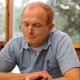# Hobsons Bay cup – round 3

Please see attached the results for l10th May and pairings for next week.

A word from our President Steve Hogan:

As advertised, juniors will start back from next week. There is therefore some additional set up time required each evening. I’ll be at the hall from around 5.30 on Tuesdays, if some people are around to assist myself and Casey with set up that will be terrific.

Round 3 results

``` 1=Beaumont,David     (2.0)-Saric,Sergey         (2.0)= 3-15 = 1-0
2=Cajes,Conrado      (2.0)-Davis,Tony J         (2.0)= 9- 4 = 0-1
3=Hogg,Dean          (1.5)-Annapureddy,Rheyansh (1.5)= 2-11 = 1-0
4=McIntyre,Julian    (1.5)-Enano,Ronald         (1.5)=16- 6 = 1-0
5=Wilkinson,Greg     (1.5)-Paul,Nikash          (1.5)=30- 8 = 0-1
6=Kirk,Ian           (1.0)-Hibberd,Nathan       (1.0)=20- 1 = 0-1
7=Szuveges,Narelle S (1.0)-Maure,Eric           (1.0)= 5-19 = 1-0
8=Galia,Aaryan       (1.0)-Hogan,Steven         (1.0)=18-10 = 0-1
9=Richards,Derek     (1.0)-Hari,Dhruv           (1.0)=13-33 = 0-1
10=Davis,Sophie       (1.0)-Matijas,Dejan        (1.0)=22-17 = 0F-1F
11=Salanowski,Mark    (0.5)-Condick,Peter        (1.0)=12-21 = 1F-0F
12=Krishnan,Anirudh   (0.5)-De Rooy,Samuel       (0.5)=14-32 = 1-0
13=Hibberd,Aaron      (0.5)-Kostakakis,Angelo    (0.5)=24-25 = ½-½
14=Waadhwa,Ehsaas     (0.0)-De Rooy,Jack         (0.0)=23-31 = ½-½
15=Mahajan,Laksh      (0.0)-Clerk,Max            (0.0)=28-26 = 0-1
16=Liu,Tina           (0.0)-Christian,Gavin      (0.0)=34-27 = 0-1
17=Santoso,Crystin    (0.0)-( bye )              (0.0)=29- 0 = 1
18=Whitford,Matthew   (1.0)-( not paired )       (0.0)= 7- 0 = 0```